﻿ Mathematical Model of Multibubble Cavitation into Sonochemical Reactor

Mathematical Model of Multibubble Cavitation into Sonochemical Reactor

Sergey D. ShestakovOPEN ACCESSPEER-REVIEWED

Mathematical Model of Multibubble Cavitation into Sonochemical Reactor

Sergey D. ShestakovThe Technological Management Institute, Moscow state university of Technologies and Management, Russian Acoustical Society, Moscow, Russia

Abstract

The research described in this paper shows that main parameter of acoustic cavitation which should be used for practical applications this phenomenon, are not the temperatures of plasma into the cavitation bubbles (the intensity of son luminescence), but the power of pressure pulses, which they produce, and which cause destruction of phases existing in a liquid (the intensity of erosion). The distribution of the density power of erosion in space can be the subject of numerically simulated, if it is assumed that process of multibubble cavitation is an ergodic process. For this the integral of pressure superposition from all bubbles of cavitation field at any point in space, must be approximated by the function of the pressure pulse on the surface of a single cavitation bubble, that pulsate with a period equal to the period of oscillations of the harmonic wave. This superposition of pressure can be described using a two metrics of space, which are belongs to this point. The first – the average distance from this a point until all points of the cavitation region. It determines the average time of arrival into this point of a total perturbation of pressure from all bubbles. The second – the average harmonic distance – determines the average coefficient of attenuation of this perturbation. The results of computational and laboratory experiments illustrate the adequacy and the applicability of model. The model makes it possible to quantitatively compare the results of physical and chemical effects of cavitation in the any liquids in the reactors of any size. The model also gives a sufficient degree of accuracy and reliability of performing the technical calculations for the design of such devices and the possibility to make comparative assessments of different reactors.

At a glance: Figures

1234
Prev Next

• Shestakov, Sergey D.. "Mathematical Model of Multibubble Cavitation into Sonochemical Reactor." American Journal of Modeling and Optimization 2.2 (2014): 60-68.
• Shestakov, S. D. (2014). Mathematical Model of Multibubble Cavitation into Sonochemical Reactor. American Journal of Modeling and Optimization, 2(2), 60-68.
• Shestakov, Sergey D.. "Mathematical Model of Multibubble Cavitation into Sonochemical Reactor." American Journal of Modeling and Optimization 2, no. 2 (2014): 60-68.

 Import into BibTeX Import into EndNote Import into RefMan Import into RefWorks

1. Introduction

Acoustic cavitation it is ripple of the vapor-gas bubbles which concentrated under the action of the ponderomotive and hydrodynamic forces in the so-called cavitation areas near antinodes of sound pressure, where it amplitude in elastic wave in the liquid exceeds a certain threshold [1, 2, 3]. They produce the cavitation noise (the secondary sound). This sound has the peak values of pressure and velocity, which are explained by the fact that waves from the bubbles have the form of shock waves due to the inertia of a dissipative dynamic system "bubble - liquid". The inertia is explained that the energy of the primary wave transformed under the action the law of conservation of momentum of pressure . Parameters of cavitation, by which determined the effectiveness of erosion and sonochemical action (of its main physical and chemical effects, which found usage at practical applications) - it is the pressure, which was achieved on the surface of bubbles , when they are compressed to minimum volume and the duration of the compression. Direct measurements parameters of compressing process are complicated due to need have of the expensive special hardware and are accompanied by methodical errors that arise when is used a majority of existing today for this methods and measurement tools. With this related the ambiguity understanding of mechanisms of liquid-phase reactions outside of the bubbles and reactions in their gas-phase, as well as accompanying of these reactions of the physical phenomena. For example, in respect of the light emission quanta, which is accompanies pyrolysis of vapor-gas mixture under the compression of the bubbles and to called sonoluminescence, there are very conflicting opinions. And this despite the fact that along with the products of plasma-chemical reactions, through the spectr of sonoluminescence are made conclusions about the dynamics of cavitation processes. The sonoluminescence is explained by thermal radiation rarely of multibubble cavitation , more often – of single-bubble , although and in her also finding differences from the spectrum of a hot body [8, 9]. The sonoluminescence not has the known practical applications, though, if it is a radiation of hot body, by virtue of the laws of Wien, Planck and Stefan-Boltzmann, she associated with the pressure on the bubbles surface and can be used to estimate of intensity of the cavitation. True, there are proposals to use her as an X-ray source  or for obtaining of thermonuclear reactions into plasma of bubbles [11-18]. In applied research directed on the cavitation effects, mainly on the erosion of solid surfaces, used the different methods of measuring and analysis of the spectra of secondary sound [19, 20], as well as the simple and available method of evaluating destructions the samples of metal, for example, the aluminum foil . And this is directly related to sonochemistry, since many from its applications, such as sonochemistry of food  are based on sonochemical reactions of the dehydration in the aqueous solutions. Reaction of dehydration - epithermal destruction of hydrogen bonds between water molecules, between the ions and water molecules as well as between the substances molecules dissolved in water and water molecules . This reaction is not accompanied by dissociation of molecules on the ions (for this is not enough of energy of the cavitation erosion, which causes destruction of substance on the intermolecular level by changing the dipole-dipole and ion-dipole interactions in this substance . It is known that water even at room temperature has a molecular structure similar to structure of ice, which too is formed by hydrogen bonds . Therefore at water and occurs epithermal reaction, caused by cavitation :(1)

where Q – heat of hydration.

Thus, the phenomenon of acoustic cavitation sufficiently is studied and described only at the level of phenomenology and empiricism. The methods of investigating the possibilities of its practical application are based on laboratory experiments and physical modeling of processes and devices. These experiments because of the ambiguity of representations of the knowledge in this area has low interpretability and reproducibility of results. The physical models it is difficult amenable "scaling"  at creation the industrial devices. Possession of the mathematical model of the cavitation and the principles of numerical comparison of the cavitation processes allows to develop applied sonochemistry and equipment without labor-intensive stages of creating a working model of industrial sonoreactors and to rely on the results of laboratory optimization. Formal description of cavitation allows to reduce the scope of research and to perform them at the expense of laboratory experiment and creation of necessary technology and equipment through a numerical simulation, based on its results. While likely absolutely to refuse from experimentation will be impossible, but it will be laboratory process which make with small amounts of ingredients and with the small-sized technique.

2. Model of Single-bubble Cavitation and the Computation Experiments with Her

Theoretical aspects of the cavitation and computation in this field are based on the differential equations of motion wall of the vapor-gas spherical cavity under the influence of an alternating external pressure [27, 28]. Analysis of variants of known equations of this kind carried out repeatedly by different researchers. For example, one of equations belongs to J.W. Strutt (Lord Rayleigh). They is also amply fulfilled in the book by R. Knapp, J. Daily and F. Hammitt. Of the equations, which most completely take into account the physical aspects of phenomenon of the cavitation, one can make the mathematical model, which is suitable for numerical analysis of the parameters of pulsating single cavitation bubble. Let the current radius of a wall of bubble R (initial diameter 2R0), the current pressure on the wall P, if the bubble is filled by a mix of a gas and a steam of liquid with an indicator of adiabaticand he pulsate under action of sinusoidal pressure, which change with amplitude A and frequency f in a liquid in densitywith viscosity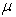and with factor of a superficial tension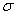. Then possible to record, by integrating system of the equations with initial conditions R = R0,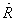= 0 :(2)

where: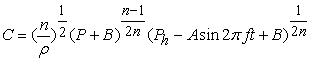– speed of sound in the liquid at the bubble wall;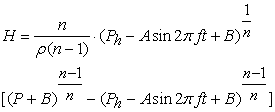– the enthalpy on the bubble wall;– derivative from an enthalpy on the bubble wall; Ph, Pv and B, n – the hydrostatic pressure in the fluid, the partial pressure of vapor and the parameters of the equation of her estate; t – dimensionless time in fractions of a period of a harmonic wave Т.

On Figure 1 and 2, are shown the results of computational experiments with a model of single-bubble cavitation, namely, in Figure 1 – which obtained by numerical decision (2) in the sets of points (size 6•104) for three randomly, selected periods of the harmonic wave:

a). Logarithms of the dimensionless pressure on bubble surface, where Р* – pressure at a time when the instantaneous value of its diameter is equal to the initial value (10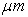), which pulsate under the influence of the elastic harmonic wave with frequency of 20 kHz, and with four different sizes of amplitude of the sound pressure in the chemically pure water with hydrostatic pressure the equal to atmospheric;

b). dimensionless pressure impulses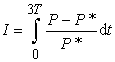– the integral characteristic, in which is included both pressure and time of its appendix ;

c). Phase trajectories on a phase plane "impulse of pressure - the logarithm of pressure" of change of the parameters of dynamical system of the bubble. On Figure 2 in the same format are shown the results of calculation of parameters of a pulsation in water of bubbles with various diameters and amplitude of sound pressure A = 5 bar.

3. Discussion of Computation Experiments with Single-bubble Model

It should be noted that the influence of viscosity of liquid on the process of pulsation of cavitation bubbles is the still not completely the deterministic of parameter of the model and along with the influence on the dynamics of process such factors as a possible vapor condensation in the bubble, heat and mass transfer through its walls and the violation of his spherical symmetry [30, 31, 32]. That may reduce the adequacy of the model. The pressure impulses on surface of a cavitation bubble during underpressure and compression in accordance with the law of conservation of energy, on average over an infinite time must be equal in magnitude, as on the front surface of wave of an underwater explosion . Otherwise, the average value over the period of oscillation of pressure in the fluid will be to infinitely increase or to fall. This will lead to spontaneous cessation of cavitation due to the full degassing the fluid, what in reality is not observed.

Figure 1. (a, b, c) The graphs of pulsation of a single bubble with initial diameter of in water at amplitudes of sound pressure: ••• – 1,25 bar; ••• – 2,5 bar; ••• – 5 bar; ••• – 10 bar with frequency 20 KHz of chemically pure water with atmospheric pressure Ph
Figure 2. (a, b, c) The graphs of pulsation of a single bubble with initial diameter: ••• – 1 m; ••• – 10 m; ••• – 100 m with the amplitude of sound pressures in water of 5 bar

On the contrary, experience shows that the acoustic cavitation in case remove heat which form by the dissipation of energy of the elastic vibrations on the internal friction in liquid possesses the property self-organizing and seeks the balance, which belongs to liquid with any amplitude of sound pressure. Therefore, in experiments the size P* – is an arbitrary parameter, and is different from the initial pressure in the bubble P0, so that his mathematical expression include also a resistance to movement of its wall, which creates a viscosity of liquid. In this case its chosen such, that phase trajectory in the phase plane (Figure 1c, Figure 2c) would have the form of limiting cycles. The experiments were executed at different sizes of the input parameters of the acoustic plane wave in the water, above which they are unlikely to be used in real conditions. For example, the maximum amplitude of sound pressure matched for intensity ultrasound 70 W/cm2. Under these conditions Р* as well as the maximum speed of compression of a bubble, depends from A and is in limits P0 < P* < A.

From analysis of the graphs on Figure 2 shows that with increasing A, the maximum value of p has increase and, obviously, reaches the limit of growth near maximal compression of bubble, which occurs at the end of half-period of compression of the harmonic wave. With further increase A at the conservation of an equal numbers of compressions and numbers of periods of the wave k, p on the average becomes equal to this limit (see Figure 1a,c and Figure 2c). The diameter of the bubble near equality of the periods of pulsation of the bubble and harmonic wave has only little influence on the form of the pressure impulse (Figure 2b).

Unfortunately, using this single-bubble model can not describe field consisting of a set of many bubbles, because she does not take into account the presence of the force field of the secondary sound. On the preparation of such equations for the whole cavitation field in the middle of last century worked the famous explorer of cavitation Professor L. Rozenberg (Acoustics Institute of the Academy of Sciences USSR), He wrote: "... even the formation of such equations - the question, which solved with enormous difficulty, not to mention about their integration. Therefore, such solution is problem of the future”. If to remember about the ergodic theory (which through the description of behavior in time of one of the elements of the force field may describe variation all this field in the geometrical space), but since a single of bubbles, which is part of the area of cavitation, is already described, it greatly simplifies task.

Analysis of single-bubble model allows us to make an important conclusion for modeling multibubble cavitation: The limit cycles of dynamics of the bubble especially near his pulsations in equal periods with the harmonic wave – are stable (see Figure 2c). And cavitation of the ensemble, consisting of a set of bubbles, which is managed by external pressure of the sound, can be considered as ergodic dynamic system. As will be shown below, this allows us to consider the distribution in space of the instantaneous parameters of the cavitation field through dynamic of single bubble over time and paves the way for writing equations of multibubble cavitation area.

4. Document Ergodic Model of Multibubble Cavitational and Computational Experiments with Her

In studies carried out under the direction of Professor Rosenberg in 1950 – 1960 was formulated the generalized approach to the estimation of energy of the elastic waves, which is dissipated on a multibubble cavitation, based on empirical data. But attempts of formalized description the fields of cavitation as functions of the spatial distribution of energy of the cavitation, began to emerge only recently [32-35]. One of them for the cavitation area acting in a standing wave with one antinode of the pressure, led to the following considerations, which make it possible to significant improvements for this model.

It is known that the erosion decreases inversely to the square of the distance from object to cavitation bubble while the attenuation of oscillatory pressure - approximately inversely to this distance [29, 30]. That is, secondary sound commits the work on the object. Measure of that work is size of energy of the destruction in the each physical point of surface object. Being divided into the duration of action, she can be named qer – the volumetric density of power of cavitation erosion (VDPCE) at this point. Thus, the problem of modeling the dynamics of the cavitation field is reduced to finding the distribution of qer in the coordinate space (x, y, z), with the beginning of coordinates, for example, which is coincide with the geometric center of the subspace v (or of the i volumes), which occupies a pulsating bubbles. To the each point of space of the cavitating liquid belong the finite number of straight lines (vectors r), which connect this point with all N bubbles. If all the bubbles have the same dimensions, then they cause instantaneous fluctuations of pressure anywhere in the cavitation region of one harmonic half-wave, which looks like on:(3)

where: r – modulus of the vector r; t - mean dimensionless arrival time of pressure fluctuations from all cavitation bubbles to the selected point.

Instantaneous value of the deviations of the pressure Р – Р* on the surface of one of the bubbles on the average for infinite time in (3), is under the sign of the two integrals (by the t and v). This is done, because the Р – Р* depends on the phase of superposition of the pressure fluctuations, which in turn depends on the linear sizes of the cavitation field. If the bubbles pulsate with periods that coincide with periods of a harmonic wave (see Figure 2), and the dimensionless pressure impulse on the surface of a single bubble is phase function, so dimensionless phase of fluctuations can be considered as the average for the geometric space superposition phase ripple across the cavitation zone at this point. It means that can be consider a dimensionless phase of pulsations how the average by geometrical space a phase of the superposition of pulsations throughout the cavitation area at a given point. Then, dropping in (3) summand R0 in denominator of the integrand of expression because of its smallness, we may write the function for mean square fluctuation of the pressure from cavitation for period of the wave at any points of space of the liquid, how:(4)

where:– the length of the harmonic wave, equal to wave length of the pressure perturbations from cavitation;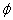– the dimensionless phase of the harmonic wave. The expression (4) can be simplified by introduction the special metrics of space. That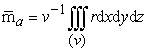and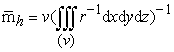– average arithmetical and average harmonical distances from a given point to all points of the cavitation region, respectively. The first, after dividing on the length of wave becomes the average time of arrival of pressure perturbations from the all bubbles of the area of cavitation to the point, for which make calculation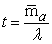. By dividing the initial radius of bubble to second metric of space, we obtain– attenuation coefficient of the total perturbations of ressure from all cavitation bubbles in this point.

Length of free run of the pressure perturbation from cavitation during the period of the harmonic wave in (4) is equal to the length of this wave. This can raise doubts as to the correctness of the interpretation (3) in connection with famous hypothesis of Kirkwood and Bethe [27, 28, 35, 36]. These doubts are eliminates Figure 3. Shown that the deviation of the absolute sound velocity near the bubble surface from the nominal occurs is very quickly and has an alternating sign. Average speed of moving into liquids of impulses of pressure from the cavitation bubbles during their pulsation, which is exceeding duration of a collapse almost in 50 times, consisting from the speed of a sound and speed of movement of a wall, remains equal to rated speed of a sound in liquid.

For an approximating the function of the pressure impulse which equal on time the period of harmonic wave in  is proposed to use piecewise linear function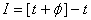, where (and further) in square brackets - is the whole part of number. Derivative of this function is the periodic generalized function of two real dimensionless variables, which in each k-th period can be expressed through δ–Dirac: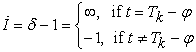(5)

Such method is similar to methods applied in the quantum mechanics where for the description of systems with the concentrated parameters also are used the generalized functions, their derivatives and integrals.

If differentiation of function of an impulse of pressure gives as result the function of a variable component of pressure upon surfaces of a bubble with a finite jump at the point of collapse, then derivative from Ip there has a point of rupture of 2-nd sort. Time of stay of a bubble in volume smaller than equilibrium volume in relation to the period of a harmonious wave is very small (see Figure 3).

Therefore, it approximation does not interfere authentically enough to describe of total impulse of pressure from cavitational area and integrate its square by phase with formation as a result of the simple expression of a kindwhere (and further) in the figured brackets - is the fractional part of number. As a result of the made transformations is possible to rewrite (3) for a cavitation in antinode of pressure in half-wave, as: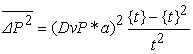(6)

where D – conditional volume density of the bubbles in liquid.

Figure 3. Graphs changes in time of: ••• – the cavitation bubble radius R; ••• – the pressure at him surface P; ••• – the speed in water on walls of babble C +in the elastic wave with amplitude of the sound pressure of 5 bar and a frequency of 20 KHz

In practice often have to deal with cavitation in the wave, which contains n antinodes of pressure and, respectively, the same number areas of cavitation. In modeling thus there arise are two problems. Solution one of them, which consists in formalization of dispersions energy of acoustic vibrations on cavitation in the plane wave, is offered for calculation of cavitation reactors in the . There the sizes of each volume vi (i = 1, 2... n) of each area is determined the square of wave front and by heights i-th of cavitation regions on her beam. Dependence of the modulus resultant amplitude of the sound pressure in the linear approximation is expressed through– coefficient of dispersions of wave energy on the cavitation ,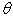– coefficient of wave reflection and A0 – amplitude of sound pressure on the surface at propagation of a ultrasound into the half-space of the liquid:(7)

for one and two identical sources of coherent waves, which are directed towards each other, respectively. The coordinates of the boundaries of volumes vi on the beam of wave in angular units are calculated as a positive roots of the transcendental equation А – Рt = 0, and their heights hi on the beam of are defined as the difference between odd and even his roots. Their converting into linear units is performed by dividing at wave number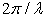.

The next problem – as in model to take into account the fact, that located next to each other on axis of the wave the cavitational area, have the opposite phase of pulsations. This problem is solved by the introducing through the metricthe correction to t, which depends on n. For antinodes of pressure, for example, with the even ordinal number the amendment to t will be equal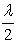. From here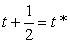, and the decision for qer is sought as the combinatorial functions . For example, if n = 2: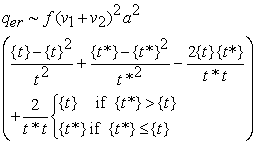(8)

Then, expressing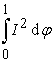at (4) as function of argumentsand n for infinite number of wave periods, regardless of initial phase, VDPCE for specific fluid can be written in the following proportions: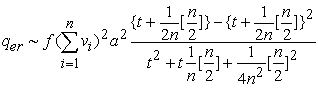(9)
Figure 4. Two-dimensional distribution VDPCE in the water in diametrical section of the reactor with top-mounted emitter 350 W in the compression phase occurring in: a). in the near area of cavitation from the transmitter; b). in the remote area of cavitation from the transmitter and c). VDPCE averaged over an infinitely large time

In the wave with amplitude of sound pressure, at which the cycles of pulsations of the bubbles occur simultaneously with periods of wave, this function allows to calculate numerically the spatial distribution VDPCE irrespective of time. Two distribution of VDPCE for period of the primary wave, calculated in accordance with (8) for specific initial the phases is shown on Figure 4 ab. One more, averaged over an infinitely large time by (9), are on Figure4c.

On Figure 5a shown the results of the evaluation the power of cavitation erosion, which are not included into paper , by the method described by R. Knapp more than half a century ago, but which frequently is used in recent studies . Figure 5b shows the distribution pattern of this VDPCE in a plane of foil the received at its computer simulation. In this case, in a special reactor with the three sources of half-waves of identical frequency, phase and intensity with the parallel beams in the plane of the antinode of sound pressure was placed sample of aluminum foil, which was subjected there to cavitation erosion.

Figure 5. The destruction from cavitation the three sources of primary coherent waves of the sample aluminum foil: a). sample foil with traces of erosion; b). mathematical model of the distribution VDPCE in the plane of foil (in the plane antinode of acoustic pressure)

General view and the scheme of the reactor, is shown in Figure 6 (is taken from ).

Figure 6. General view and schematic diagram of the experimental reactor to study the effect of nonparametric amplification of cavitation: 1 – photomultiplier tube; 2 – hydrophone; 3, 5 – amplifiers; 4, 6 – integrators; 7 – device of strobing; 8 – device of synchronization; 9 – delay line; 10... 15 – ultrasonic generators; 16 – sample foil

Figure 7a also shows the results of an experiment of erosion of the aluminum foil in the reactor which has height equal one wave, one ultrasonic emitter and one reflector, and Figure 7b the result of computer simulation of the distribution the relative VDPCE in this case. The sample has been established, as shown by dotted line, in parallel to a wave beam in volume of the laboratory reactor .

Figure 8a is shown a design of the reactor with a symmetric vibration system of the acoustic cell, made with glass wall, created by Estonian company "Oil Tech Production OY" . Figure 8b is shown a mathematical model of distribution VDPCE in the diametric plane of the body of the reactor. It is clearly seen that the distribution corresponds to a visual picture of cavitation.

Figure 7. Cavitational destruction the aluminum foil: a). the erosion of sample foil; b). mathematical model of the distribution VDPCE relative to the plane of the foil
Figure 8. Reactor with symmetric vibrational system of the acoustic cell, made in a glass body (а); A mathematical model of the distribution VDPCE (b)

5. Discussion of Results of the Computational Experiments with the Model of Multibubble Cavitation. The Hypothesis of the Similarity of Cavitation Processes

In comparative experiments (Figure 5) was utilized sources of ultrasound from the processor UIP-250 German-made with waveguides for the amplitude of sound pressure in the water 20 bar  and 2 bar  (Figure 7). However, the destruction of the aluminum foil caused by cavitation in three coherent half-waves, and also in the two areas of cavitation of one wave, are a clear demonstration of sufficient adequacy of the model, even though period of sound wave and periods of oscillation of cavitation bubbles can be and not coinciding. Comparison of this destruction of the foil with the distribution of the VDPCE shows that the model is applicable even in the presence of several independent waves in the liquid, if the oscillations are the coherent. By phenomenological description of this model can explain fact of the dependence of pressure, achieved on collapse of a bubble from the size of the all cavitation field which is established, for example, in [16, 38]. The parameters N or D, R0 and P* – the constants, that characterize a specific liquid and its behavior in cavitation process. Therefore, if in some way become known and used in the calculation their sizes, obtained in absolute units for particular fluid, the numerical results of its can be applied only to this fluid. In practice, the study of any applications of cavitation usually starts with a practical process optimization in the laboratory. To enlarge its the scope to industrial use sonotechnology suitable for industrial process first required make the prototype of industrial equipment or, using the similarity of cavitation processes for different sizes, make a numerical model of industrial technology, the results of which then will be used for design the industrial devices, bypassing the stage of prototype. It is clear that the second option makes the project much cheaper, and the model described above allows implementing it.

Since with such a strategy the implementation of the project in the research lab and on production the liquids are one and the same, to select the optimal acoustic parameters and required absolute sizes for the industrial reactors, knowing these characteristics for devices, which were used for optimization in the laboratory, one can use the model. For example, result of chemical process in the laboratory reactor with working volume Vlab, which has some size of average VDPCE and its distribution in this volume, achieved the optimum productivity. It can be assumed that in the reactor with arbitrarily chosen volume and with other conditions of wave formation, but the same dispersion of distribution VDPCE in the same liquid can be reached a productivity, which differs in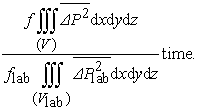(10)

The equal constants in this expression and units of dimensions of other variables in the numerator and denominator cancel each other and in result is obtained a dimensionless parameter – the ratio of productivity of the reactors, for example, for newly developed reactor and for laboratory reactor with known technical characteristics (the need for energy, the sizes, the weight etc.). This makes it possible to compare them with each other in processing of liquids with the same parameters.

6. Experimental Confirmation of the Theory of Similarity of Cavitation Processes and the Possibility of Optimization of the Sonochemical Technologies

The practical test of this method of comparison of the cavitation processes is carried out on well-known effect of dispersing the phases of aqueous sols. As the experimental setup (Figure 9), where is used reactor with the casing of working volume, made of standard stainless seamless pipe, which has two channels for flow of sol. At the ends of this casing through O-rings are mounted two acoustic transformers that transmit fluctuations of the primary sound from emitters to liquid. Their waves are coherent. The distance between their emitting surfaces is equal to 1,5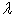. Were used two variants of the device. In the first embodiment, when has been the work, each of the sources was turned on. In the second embodiment, bottom source (Figure 9) always turned off. His waveguide play the role of the reflector. Electrical power is served only to upper source.

Figure 9. The design of the experimental reactor. In the axial sections are shown VDPCE distributions. left - in the first embodiment, on the Right - in the second

To dispersing was subjected the sol of polystyrene latex which was obtained by emulsion polymerization of styrene, with average diameter of sphere of latex particle near 300 nm. In reactor the cavitation erosion commits a work against the integrity of the particles of polystyrene. In the experiment, five samples of latex with the equal initial optical density passed through the reactor with the equal initial temperature. Then, by a monochrome turbidimeter on the wavelength 400... 600 nm with a resolution of 20 nm determined optical transmittance of samples sols. Transverse sizes of the particles was calculated from the angles of slope of the graphs of the logarithms of optical density, assuming the form of particles the spherical with using the known empirical equation of Geller. The results of dispersing particles of latex is shown in Table 1.

The experiment confirms an attitude 1.92, obtained from the model. In experiment with a near 90% probability it is in a range 1.67...2.16. That is, for the chosen quantitative method of testing the correctness of expression (10) the result is quite satisfactory.

Table 1. The results of the experiment on dispersibility of polystyrene latexDownload asPowerPoint Slide

Veiw figureView current table in a new window

With using the developed model was implemented optimization costs of energy on desintegration of water when hydrated milk protein for increase of dispersion fat globules in the milk (the increase viscosity of milk). Optimization was carried out using of the reactor 1 shown in Figure 9 (left) in recirculation mode on the stand (Figure 10), consisting of the dispersant 2, in as was which used a laboratory pump-emulsifier, of the mixer 3, filled before each experiment of the whole milk by three quarters. The feed rate of water into the mixer is always maintained constant. For this used stabilizer 4, consisting of a coaxially placed one inside the other tanks and throttle 5 which is set on leaving out of them and is opened on minimum flow rate. Skimmed milk powder is introduced into the mixer. Into the internal tank of the stabilizer from the outlet of the reactor through a three-way valve 6 is continuously fed water after treatment. Performance adjusted through the throttle 7 setting on the output of the reactor. Thus, the reactor can run with any productivity, but the water from the stabilizer into the mixer is always fed at the same rate. Excess water at the filling of the tank flows through the drain pipe 8 which mounted on the bottom of the outer container. In the mixer, which is being filled during each experiment to the mark 9 for effective the mechanical agitation was set agitator 10. That is, mixing container had the appearance tank for pasteurizing or fermenting which used in dairy industry.

Figure 10. Experimental stand for practical optimization: 1 - reactor; 2 - dispersant; 3 - mixer; 4 - stabilizer; 5 - throttle; 6 - three-way valve; 7 - throttle; 8 - drain pipe; 9 - mark; 10 - agitator

The mixer was included in the scheme of the recirculation through a dispersant of mixture with a given speed. The experiment was conducted at room temperature. Five samples of mixtures were prepared with different productivities of the reactor. Into the mixer is strewed in accordance with  skimmed milk powder, then include the agitator of mixer, include the dispersant and through 5 seconds is switching the three-way valve on the supply of processed water in stabilizer. By filling out the mixer to reach mark 9 three-way valve switch is set for discharge of water through the drain pipe, the reactor to off, and mixing and dispersing are continued for another 20 minutes. The viscosity was measured by rotational viscometer in the volumes taken from the central parts of the tanks for storage of samples for three days at a temperature +8°C. The results of these measurements were divided by the viscosity of whole milk with the same number of skimmed milk powder and the water, but without sonochemical processing. The received discretes depending of the relative viscosity on the speed of processing were approximated to polynom a second-order the method of least squares. The abscissa of point corresponding the performance 0.12 m3/h which lying on the branch of a parabola on the other side from the maximum is equals 0.3 m3/h. Thus obtained the range of viscosity of milk and, consequently, the dispersion of his fat phase, more than at in . That diapason is corresponds to diapason of specific energies of water processing 5...9 MJ/m3 which can be prepared from  according to formula (10).

7. Conclusions

The above described mathematical model is considering only deterministic component of a formalized description of the cavitation process, since equality of frequency of the primary wave and of oscillations of bubbles requires a complete identity of their diameters. But in real liquids there is always distribution of bubbles by their initial diameters. This requires the introduction of a stochastic component of the model.

The evaluation basing on model also will be always relative and will remain such, pending the availability of methods and tools that can accurately determine the absolute values of the parameters in (10) which were eliminated by dividing each on other, because they belong one liquid. Yet this model has real practical value. The theory of similarity of the cavitation processes makes it possible to design reactors, basing on the results of laboratory optimization of these processes.

On the basis of this theory one can provide practical optimization of industrial sonochemical the technologies and to find optimal parameters of processes with minimum number of experiments.

In addition, model has a predictive function, which consists into simple and rapid a comparative evaluation of the effectiveness of the existing acoustic devices to apply them in area of cavitation technology. All it gives her the right to exist.

References

  Mettin R., et al., 1997. Bjerknes forces between small cavitation bubbles in a strong acoustic field. Phys. Rev. 56, 3, 2924-2931.In article  Mettin R., Koch Ph., & Lauterborn W., 2006. Modeling acoustic cavitation with bubble redistribution 6-th International Symposium on Cavitation, Wageningen.In article  Shestakov S., 2001. The basic technology of cavitation disintegration. Mоskow: EVA-Press (in Russian).In article  Shestakov S., 2009. Management of hydration the food biopolymers. In V. Panfilov (Eds.). Theoretical Foundations of Food Technology. Moskow: ColosS, (in Russian).In article  Margulis M., 1997. Patent RU 2096934.In article  Dezhkunov N., et al., 2000. Enhancement of sonoluminescence emission from a multibabble cavitation zone. Ultrasonics Sonochemistry, V 7, 1, 19-24.In article CrossRef  Margulis M., 2000. Sonoluminescence. Physics-Uspekhi, 170, 263-287 (in Russian).In article  Matula T., et al., 1995. Comparison of Multibubble and Single-Bubble Sonoluminescence Spectra. Phys. Rev. Lett., 75, 2602-2605.In article CrossRef  Flannigan D., Suslik K., 2005. Plasma formation and temperature measurement during single-bubble cavitation. Letters to Nature, 434, 52-55.In article CrossRef  Shestakov S., 2008. Research of an opportunity to strengthen the nonparametric multibubble cavitation. Applied Physics, 6, 18-24 (in Russian).In article  Flynn H., 1982. Patent US 4333796.In article  Putterman S. et al., 1994. Patent US 5659173.In article  Taleyarkhan R. et al., 2002. Evidence for Nuclear Emissions During Acoustic Cavitation. Science, V. 295, 1868-1873.In article CrossRef  Taleyarkhan R. et al., 2004. Additional evidence of nuclear emissions during acoustic cavitation. Physical Review, V. 69, 036109.In article  Nigmatulin R., 2005. Nano-scale thermonuclear fusion in imploding vapor bubbles. Nuclear Engineering and Design, V. 235, 1079-1091.In article CrossRef  Lahey R., Taleyarkhan R., & Nigmatulin R., 2007. Sonofusion technology revisted. Nuclear Engineering and Design, V. 237, 1571-1585.In article CrossRef  Khavroshkin O., Bystrov V., 2007. Sonoluminescence and Sono-fusion. Applied Physics, 5, 7-14 (in Russian).In article  Shestakov S., 2007. Patent EP 1810744.In article  Dezhkunov N., Ignatenko P., & Kotukhov A., 2007. Optimization of the activity of cavitation generated by pulsed ultrasound. Electronic Journal “Technical Acoustics”. http://www.ejta.org, 2007, 16 (in Russian).In article  Lanin V., Dezhkunov N. & Tomal V., 2008. Instrumentation for measurement of ultrasonic effects in processes. Technology and design of electronic equipment, 2, 51-55 (in Russian).In article  Krefting D., Mettin R. & Lauterborn W., 2004. High-speed observation of acoustic cavitation erosion in multibubble systems. Ultrasonics Sonochemistry, 11, 119-123.In article CrossRef  Shestakov S., & Krasulya O., 2010. Sonochemical technologies in food industry. Electronic Journal “Technical Acoustics”, http://www.ejta.org, 2010, 10 (in Russian).In article  Rogov I., & Shestakov S., 2004. Epithermal change the thermodynamic equilibrium of water and aqueous solutions: Delusion and Reality. Storage and Processing of Farm Products, 4, 17-20; 10, 9-13 (in Russian).In article  Ashokkumar M., Rink R., & Shestakov S., 2011. Hydrodynamic cavitation – an alternative to ultrasonic food processing. Electronic Journal “Technical Acoustics”. http://www.ejta.org, 2011, 9.In article  Jinesh K. & Frenken J., 2008. Experimental Evidence for Ice Formation at Room Temperature. Physical Review Letters, 101, 036101.In article CrossRef  Mawson R., & Knoerzer K., 2007. A brief history of the application of ultrasonics in food processing. 19-th ICA Congress, Madrid.In article  Knapp R., Daily J., & Hammitt F., 1970. Cavitation. NY: McGraw Book Company.In article  Rozenberg L. (Eds.), 1968. Physics and technology of high-intensity ultrasound. Moskow: Nauka, (in Russian).In article  Podobriy G. M. et al., 1969. Theoretical foundations of torpedo weapons. Moscow: Military (in Russian).In article  Klotz A., & Hynynen K., 2010. Simulations of the Devin and Zudin modified Rayleigh-Plesset equations to model bubble dynamics in a tube. Electronic Journal “Technical Acoustics”, http://www.ejta.org, 2010, 11.In article  Melnikov P., Makarenko V. & Makarenko M., 2004. Achievement of high temperatures during compression vapor bubble. J. of Appl. Mechanics and Tech. Physics, V 45, 4, 13-25 (in Russian).In article  Gaitan D., Tessien R., & Hiller R., 2007. Pressure pulses from transient cavitation in high-q resonators. 19-th ICA Congress, Madrid.In article  Mettin R., Koch Ph. & Lauterborn W., 2006. Modeling acoustic cavitation with bubble redistribution 6-th International Symposium on Cavitation, Wageningen.In article  Shestakov S., & Befus A., 2008. The formulation of the criterion of similarity sonochemical reactors processing environments that do not ensure acoustic resonance. Dep. VINITI, 840-B2008 (in Russian).In article  Lavrinenko O., Savina E. & Leonov G., 2007. Modeling mechanical-physical and chemical effects in the collapse of cavitation bubbles. Polzunov Bulletin, 3, 59-63 (in Russian).In article  Kedrinskiy V., 1975. Dynamics of the cavitation zone at underwater explosion near free surface. J. of Appl. Mechanics and Tech. Physics, 5, 68-78 (in Russian).In article  Rink R. & Shestakov S., 2012. Cavitational reactor with symmetric nonmonolithic oscillatory system of the acoustic cell for processes of food sonochemistry. Electronic Journal “Technical Acoustics”, http://www.ejta.org, 2012, 2.In article  Mettin R., et al., 1997. Bjerknes forces between small cavitation bubbles in a strong acoustic field. Phys. Rev. 56, 3, 2924-2931.In article  Patent application WO 2007111524, 2007.In article